﻿ HOI延迟对BDS对流层参数估计的影响文章快速检索 高级检索
 大地测量与地球动力学2023, Vol. 43Issue (9): 893-898  DOI: 10.14075/j.jgg.2023.09.003### 引用本文FANG Zhuo, WEI Tong, FANG Hairuo, et al. Effects of the HOI Delay on BDS-Based Tropospheric Parameter Estimations[J]. Journal of Geodesy and Geodynamics, 2023, 43(9): 893-898.### 第一作者简介

FANG Zhuo, assistant engineer, majors in satellite navigation and positioning technology, E-mail:1755877893@qq.com.

### 文章历史

HOI延迟对BDS对流层参数估计的影响

1. 南京数维测绘有限公司，南京市砖墙经济园367号，211808;
2. 沈阳建筑大学交通与测绘工程学院，沈阳市浑南中路25号，110168;
3. 中北大学材料科学与工程学院，太原市学院路3号，030051;
4. 南京农业大学公共管理学院，南京市卫岗1号，210095

1 数据与方法 1.1 测站选择

1.2 算法原理

 $\left\{\begin{array}{l} {\mathit{\Phi}}_{L i}=\rho^{\prime}+\mathrm{ZTD}-\mathrm{HOI}+c\left(\delta t_{\mathrm{r}}-\delta t^{\mathrm{s}}\right)+ \\ \quad \lambda N_{L i}+\alpha_{\mathrm{r}}-\alpha^{\mathrm{s}}+v_{\Phi_{L i}} \\ \mathrm{PR}_{L i}=\rho^{\prime}+\mathrm{ZTD}+\mathrm{HOI}+ \\ \quad c\left(\delta t_{\mathrm{r}}-\delta t^{\mathrm{s}}\right)+d_{\mathrm{r}}-d^{\mathrm{s}}+v_{\mathrm{PR}_{L i}} \end{array}\right.$ (1)

 $I_{d, L i}^{(2)}=\frac{e A}{f_{L i}^3 2 \pi m_{\mathrm{e}}}\|\boldsymbol{B}\||\cos \theta| \mathrm{TEC}$ (2)

 $I_{d, L i}^{(3)}=\frac{3 A^2}{8 f_{L i}^4} \eta N_{e, \max } \text { TEC }$ (3)

 $\begin{gathered} \mathrm{TEC}=\frac{f_{L 1}^2 f_{L 2}^2}{40.3\left(f_{L 1}^2-f_{L 2}^2\right)} \cdot \\ \left(\mathrm{PR}_{L 1}-\mathrm{PR}_{L 2}-c\left(\mathrm{DCB}_{\mathrm{r}}+\mathrm{DCB}_{\mathrm{s}}\right)+\varepsilon_{L 1 L 2}\right) \end{gathered}$ (4)

 $\begin{gathered} T_d=T_{\mathrm{W} d}+T_{\mathrm{D} d}=T_{\mathrm{ZW} d} \mathrm{MF}_{\mathrm{wet}(\varepsilon)}+ \\ T_{\mathrm{ZD} d} \mathrm{MF}_{\mathrm{dry}(\varepsilon)}+A_{d(\varepsilon, \alpha)} \end{gathered}$ (5)

 $\begin{gathered} A_{d(\varepsilon, a)}=\mathrm{NS}_{\mathrm{grad}} \cos \alpha \mathrm{MF}(\varepsilon)+\mathrm{EW}_{\mathrm{grad}} \sin \alpha \mathrm{MF}(\varepsilon), \\ \mathrm{MF}_{\varepsilon}=\frac{1}{\sin \varepsilon \tan \varepsilon+0.0032} \end{gathered}$ (6)

 $\boldsymbol{X}=\left(\overline{\mathrm{ZTD}}, \mathrm{NS}_{\mathrm{grad}}, \mathrm{EW}_{\mathrm{grad}}\right), \overline{\mathrm{ZTD}}=T_{\mathrm{ZW} d}+T_{\mathrm{ZD} d}$ (7)
2 算例分析 2.1 太阳活动低水平时期表 1 太阳活动低水平时期状况 Tab. 1 Status of low solar activity
2.1.1 HOI对对流层ZTD和梯度估计的影响表 2 ZTD差值mean和RMS Tab. 2 Mean and RMS values of the ZTD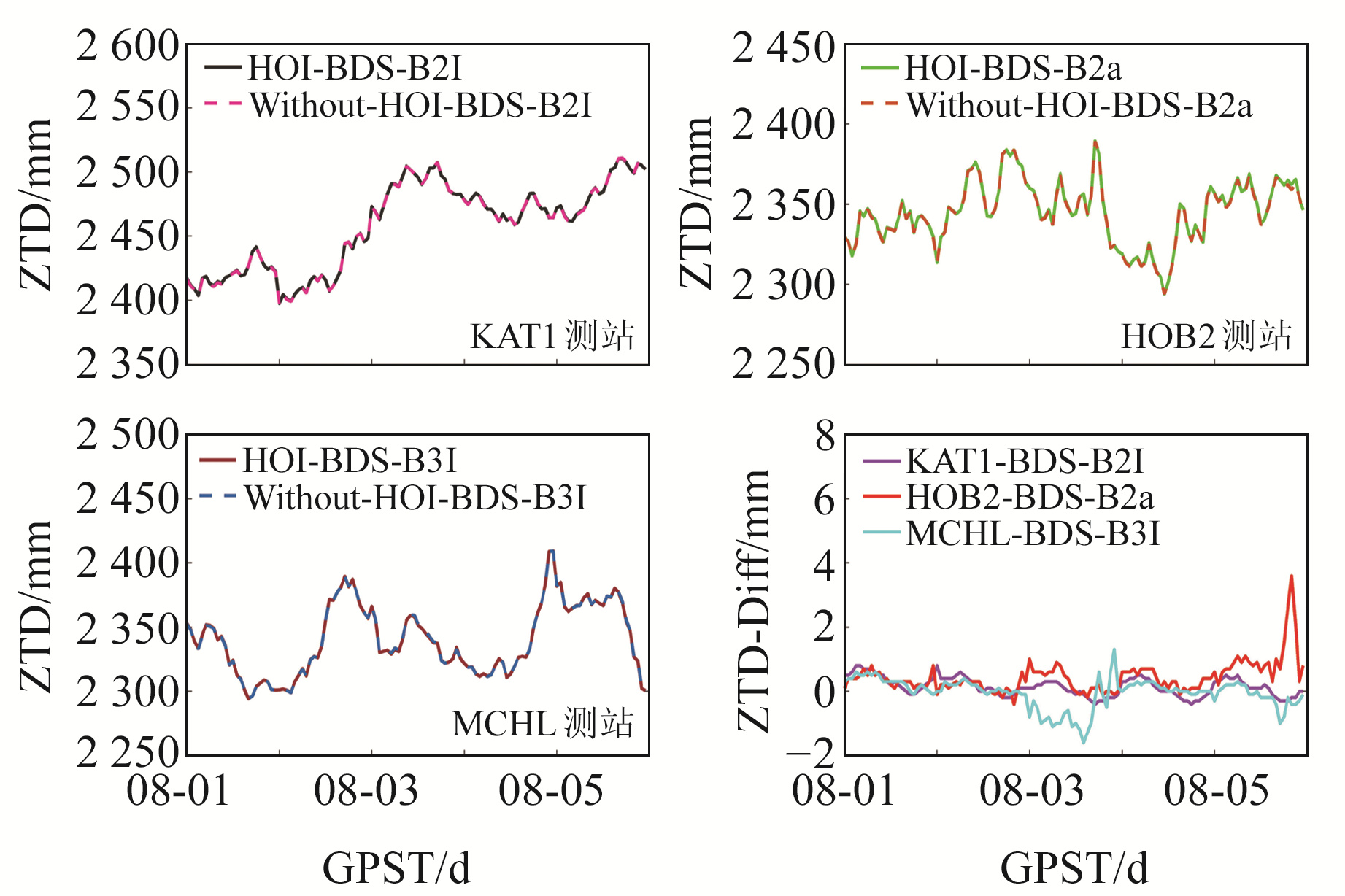图 1 ZTD和ZTD差值时间序列 Fig. 1 Time series of the ZTD and ZTD differences表 3 南北和东西梯度差值mean和RMS Tab. 3 Mean and RMS values of the NS and EW gradients differences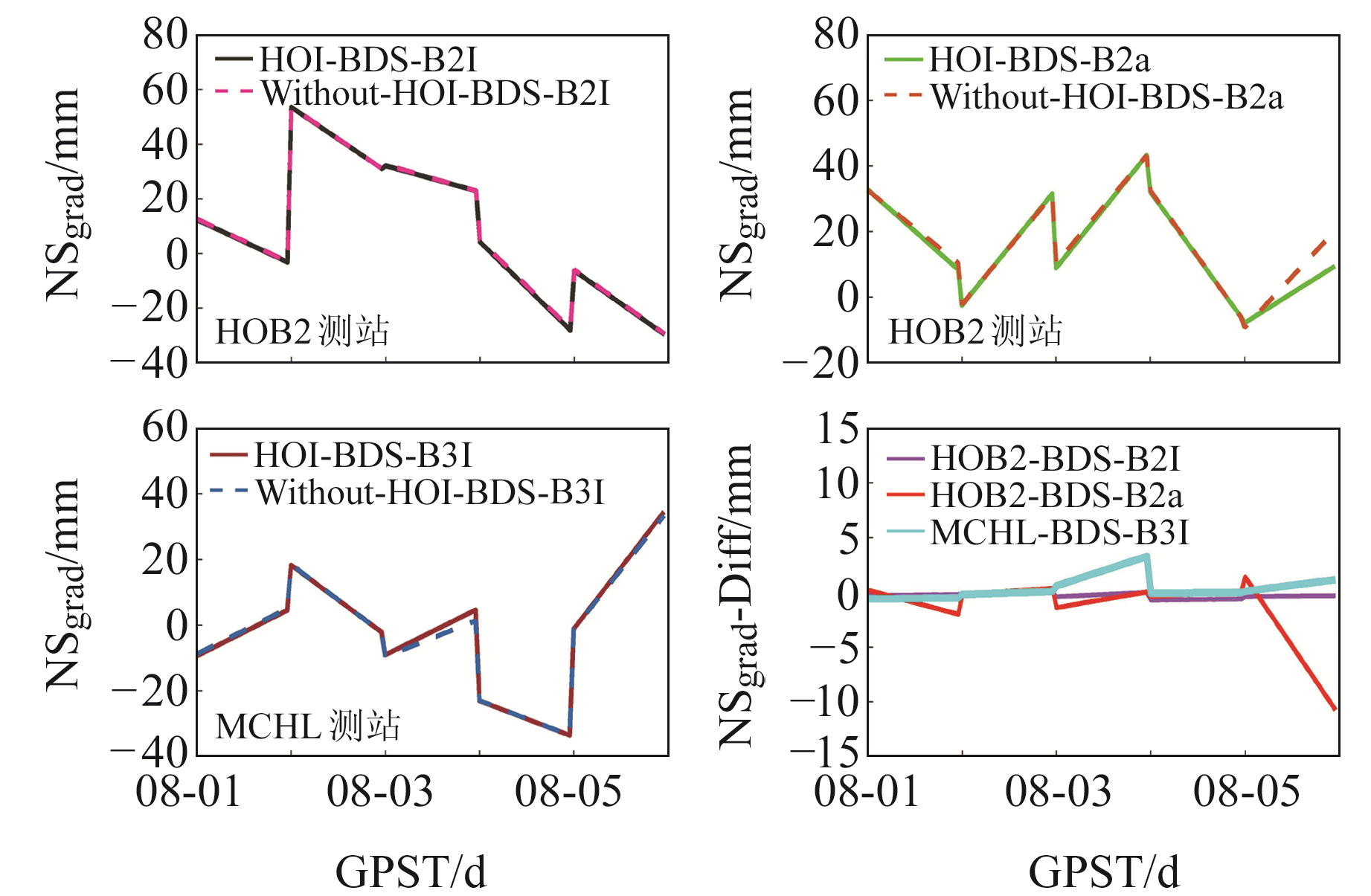图 2 NSgrad和NSgrad差值时间序列 Fig. 2 Time series of the NS gradients and NS gradients differences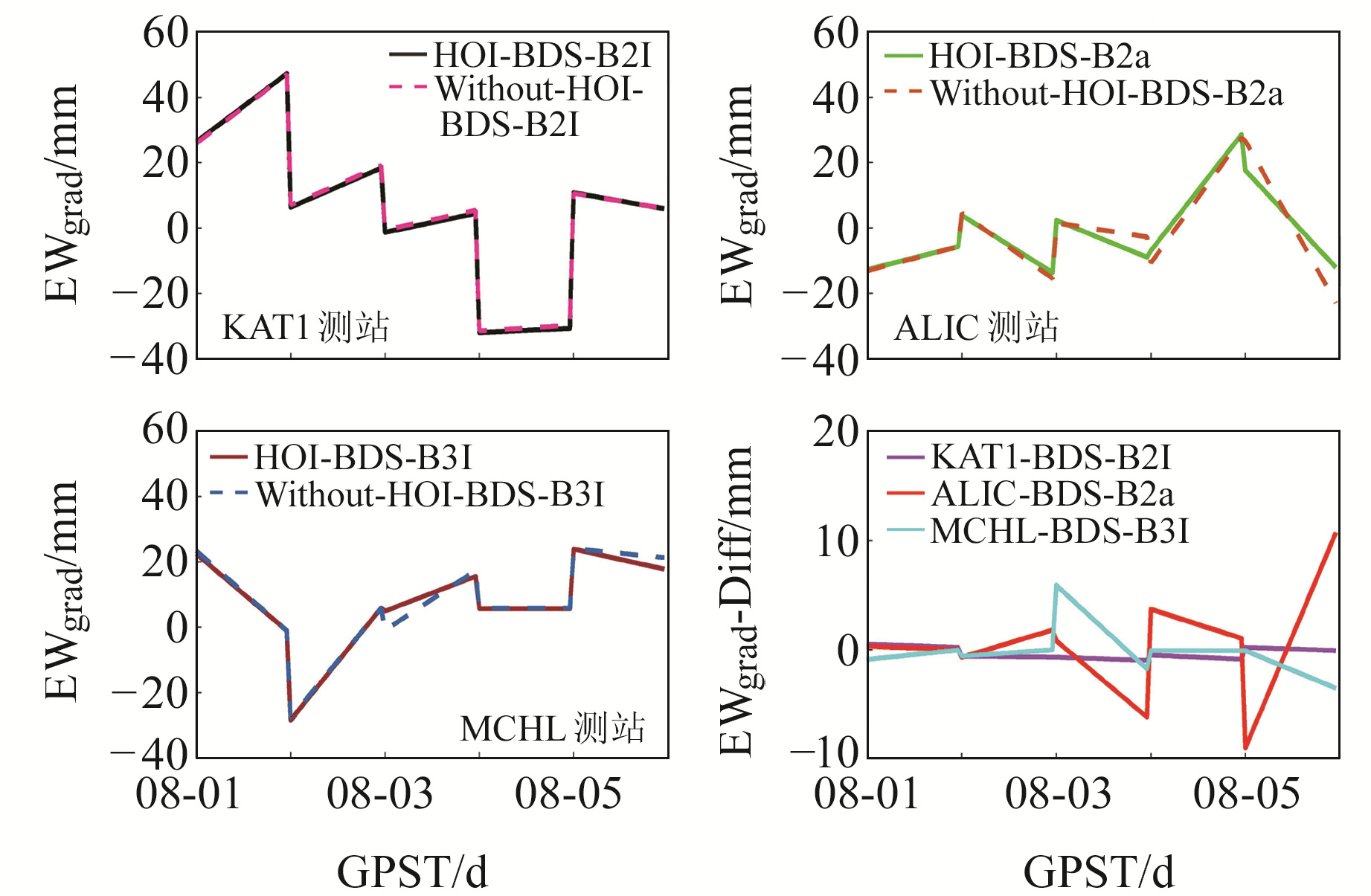图 3 EWgrad和EWgrad差值时间序列 Fig. 3 Time series of the EW gradients and EW gradients differences

2.1.2 HOI在昼夜对流层ZTD估计中的影响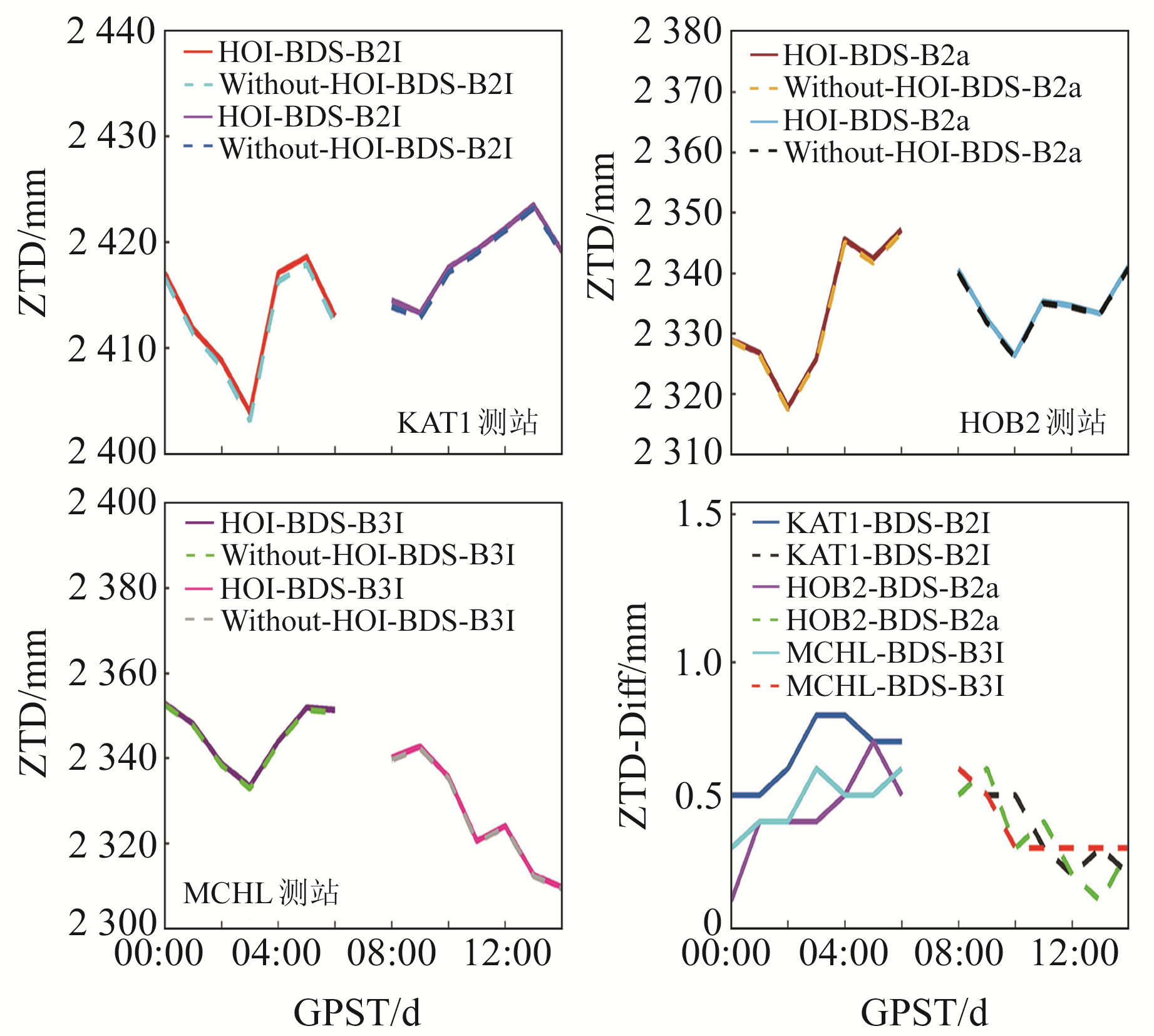图 4 2022-08-01 ZTD差值时间序列 Fig. 4 Time series of the ZTD differences on August 1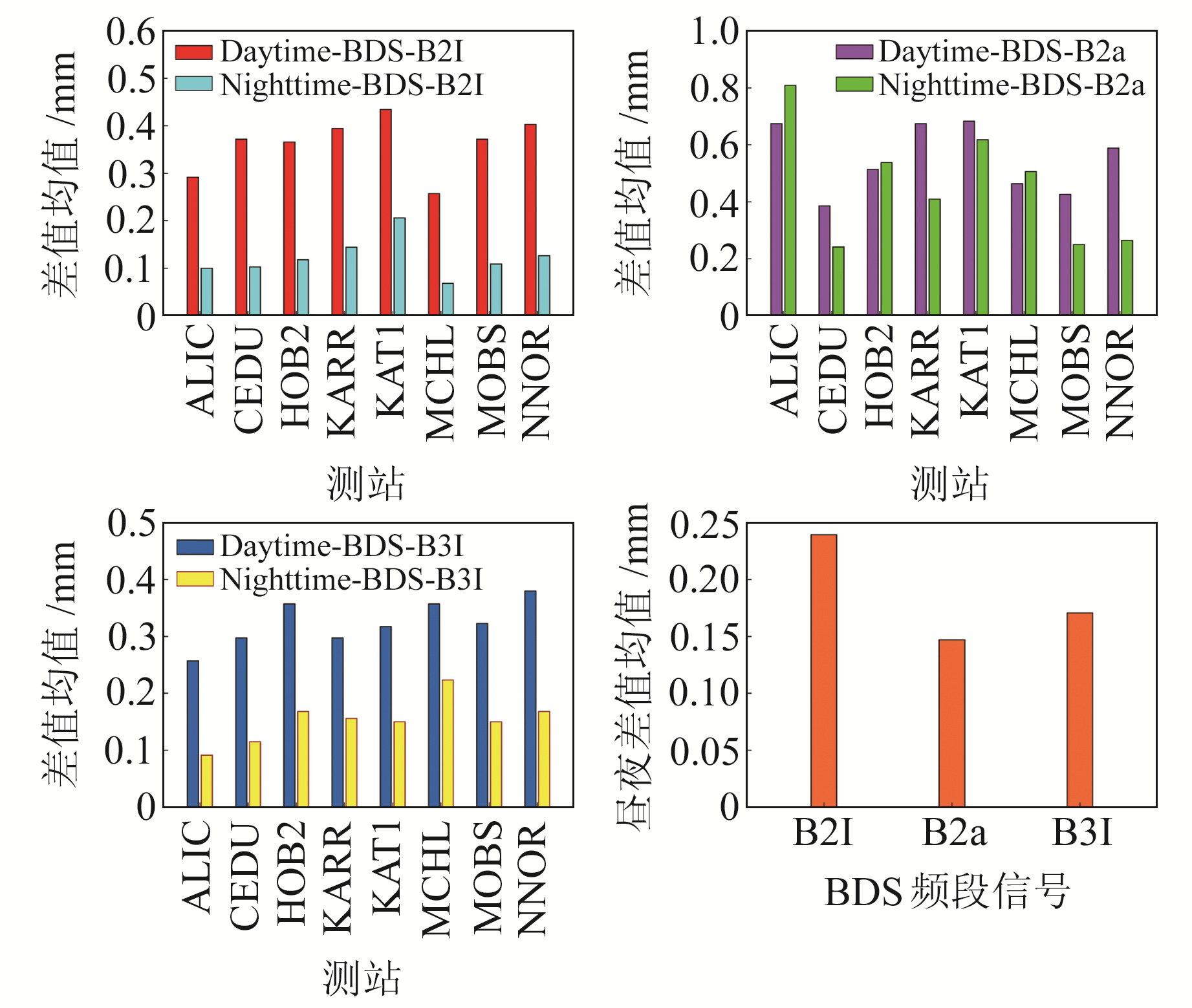图 5 MGEX跟踪站昼夜ZTD差值均值 Fig. 5 Daytime and nighttime ZTD differences mean of MEGX tracking stations

2.2 太阳活动高水平时期表 4 太阳活动高水平时期状况 Tab. 4 Status of high solar activity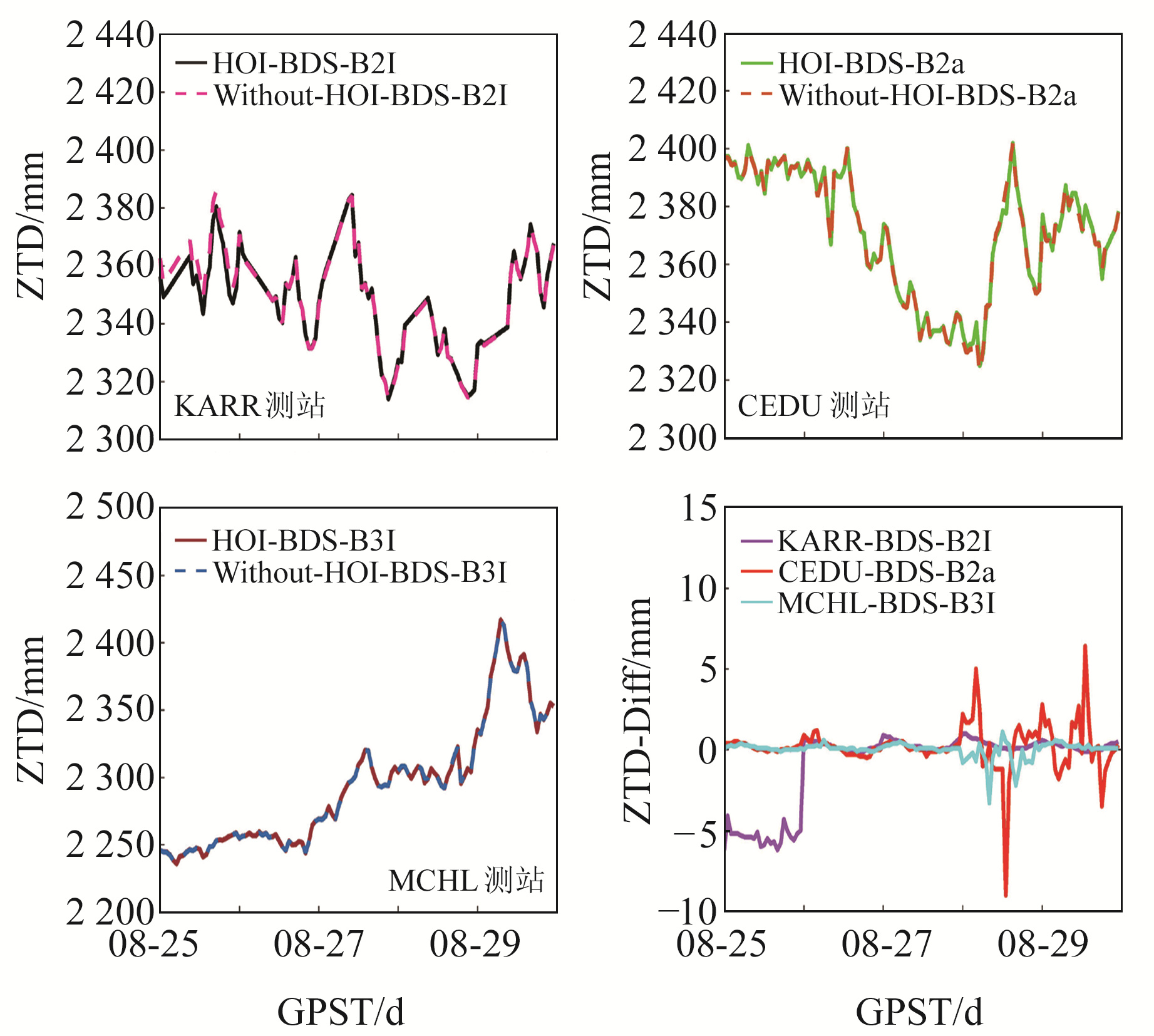图 6 ZTD和ZTD差值时间序列 Fig. 6 Time series of the ZTD and ZTD differences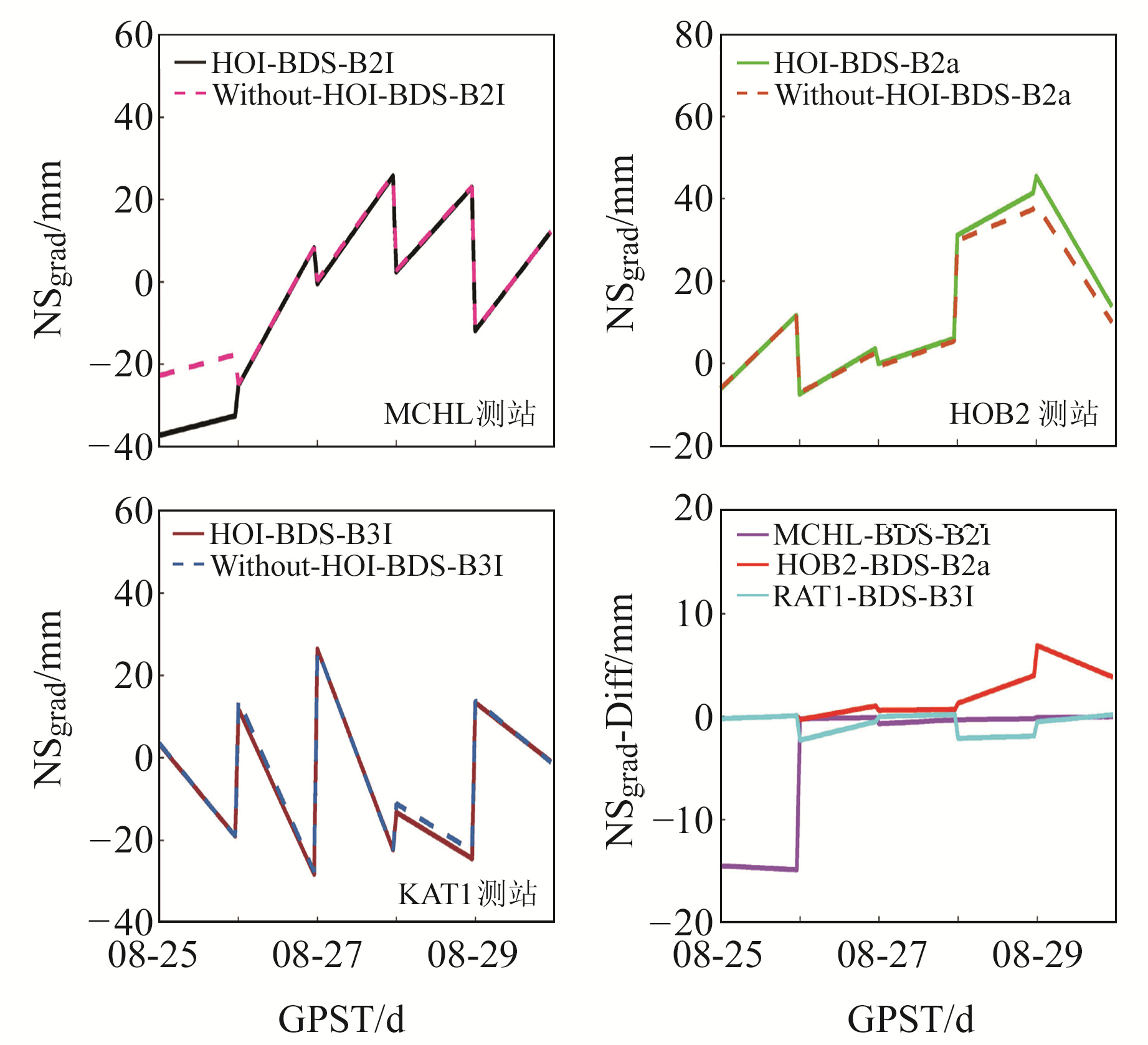图 7 NSgrad和NSgrad差值时间序列 Fig. 7 Time series of the NS gradients and NS gradients differences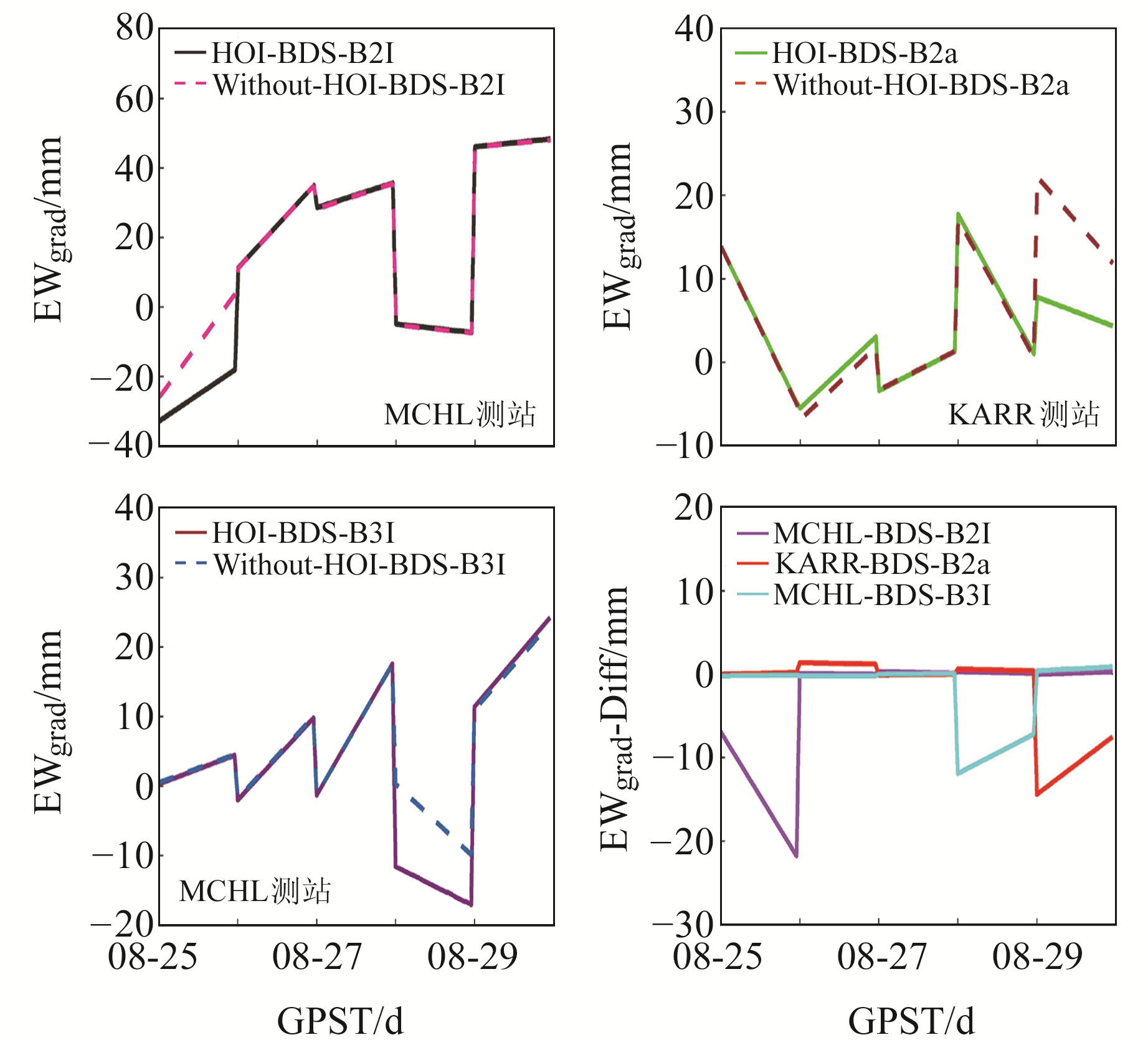图 8 EWgrad和EWgrad差值时间序列 Fig. 8 Time series of the EW gradients and EW gradients differences

3 结语

  Bevis M, Businger S, Herring T A, et al. GPS Meteorology: Remote Sensing of Atmospheric Water Vapor Using the Global Positioning System[J]. Journal of Geophysical Research, 1992, 97(D14) (0)  Bevis M, Businger S, Chiswell S, et al. GPS Meteorology: Mapping Zenith Wet Delays Onto Precipitable Water[J]. Journal of Applied Meteorology, 1994, 33(3): 379-386 DOI:10.1175/1520-0450(1994)033<0379:GMMZWD>2.0.CO;2 (0)  Businger S, Chiswell S R, Bevis M, et al. The Promise of GPS in Atmospheric Monitoring[J]. Bulletin of the American Meteorological Society, 1996, 77(1): 5-18 DOI:10.1175/1520-0477(1996)077<0005:TPOGIA>2.0.CO;2 (0)  Yuan Y B, Zhang K F, Rohm W, et al. Real-Time Retrieval of Precipitable Water Vapor from GPS Precise Point Positioning[J]. Journal of Geophysical Research: Atmospheres, 2014, 119(16): 10 044-10 057 DOI:10.1002/2014JD021486 (0)  Gurbuz G, Jin S G. Long-Time Variations of Precipitable Water Vapour Estimated from GPS, MODIS and Radiosonde Observations in Turkey[J]. International Journal of Climatology, 2017, 37(15): 5 170-5 180 DOI:10.1002/joc.5153 (0)  Rocken C, Van Hove T, Johnson J, et al. GPS/STORM-GPS Sensing of Atmospheric Water Vapor for Meteorology[J]. Journal of Atmospheric and Oceanic Technology, 1995, 12(3): 468-478 DOI:10.1175/1520-0426(1995)012<0468:GSOAWV>2.0.CO;2 (0)  Jin S, Park J U, Cho J H, et al. Seasonal Variability of GPS-Derived Zenith Tropospheric Delay(1994-2006) and Climate Implications[J]. Journal of Geophysical Research: Atmospheres, 2007, 112(D9) (0)  Jin S G, Li Z, Cho J. Integrated Water Vapor Field and Multiscale Variations over China from GPS Measurements[J]. Journal of Applied Meteorology and Climatology, 2008, 47(11): 3 008-3 015 DOI:10.1175/2008JAMC1920.1 (0)  Jin S G, Luo O F. Variability and Climatology of PWV from Global 13-Year GPS Observations[J]. IEEE Transactions on Geoscience and Remote Sensing, 2009, 47(7): 1 918-1 924 DOI:10.1109/TGRS.2008.2010401 (0)  Cai C S, Liu G, Yi Z H, et al. Effect Analysis of Higher-Order Ionospheric Corrections on Quad-Constellation GNSS PPP[J]. Measurement Science and Technology, 2019, 30(2) (0)  Zhou H T, Wang L, Fu W, et al. Impact of Higher-Order Ionospheric Delay on the Reliability of RTK Ambiguity Estimation[J]. Advances in Space Research, 2022, 69(1): 727-736 DOI:10.1016/j.asr.2021.09.031 (0)  曹炳强, 成英燕, 许长辉, 等. 间距分区法在解算卫星连续运行站数据中的应用[J]. 测绘通报, 2016(11): 15-17 (Cao Bingqiang, Cheng Yingyan, Xu Changhui, et al. Application of Space Partition Method in the CORS Data Processing[J]. Bulletin of Surveying and Mapping, 2016(11): 15-17) (0)
Effects of the HOI Delay on BDS-Based Tropospheric Parameter Estimations
FANG Zhuo1WEI Tong2FANG Hairuo3     ZHANG Bingliang4
1. Nanjing Shuwei Survey Co Ltd, 367 Brick Wall Economic Park, Nanjing 211808, China;
2. School of Transportation and Geomatics Engineering, Shenyang Jianzhu University, 25 Mid-Hunnan Road, Shenyang 110168, China;
3. School of Materials Science and Engineering, North University of China, 3 Xueyuan Road, Taiyuan 030051, China;
4. College of Public Administration, Nanjing Agricultural University, 1 Weigang, Nanjing 210095, China
Abstract: Based on GAMIT10.71, we study the influence of high-order ionosphere (HOI) delay on the estimation of tropospheric parameters of B2I, B2a and B3I frequency signals of Beidou navigation satellite system(BDS). The experimental results show that during low levels of solar activity, the maximum influence of HOI delay on the zenith total delay(ZTD), north south gradient(NSgrad) and east west gradient(EWgrad) of B2I is 0.80 mm, 0.60 mm and 0.99 mm respectively. The maximum influence of ZTD, NSgrad and EWgrad of B2a is 3.60 mm, 10.77 mm and 10.74 mm respectively. The maximum impact of ZTD, NSgrad and EWgrad of B3I is 1.60 mm, 3.28 mm and 5.90 mm respectively. During the period of high solar activity, HOI has a greater impact on the estimation of tropospheric parameters. The experimental results further show that the influence of HOI on tropospheric parameter estimation is higher in the daytime than at night, and the influence of HOI in low latitude is higher than that in high latitude.
Key words: high-order ionosphere (HOI) delay; frequency band signal; low level of solar activity; high level of solar activity; tropospheric parameter estimation